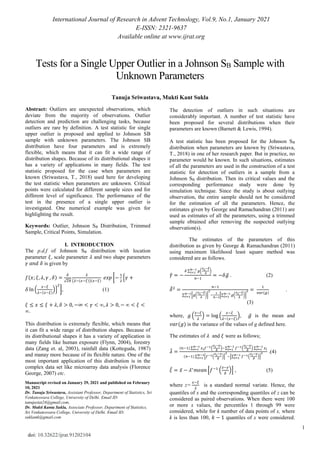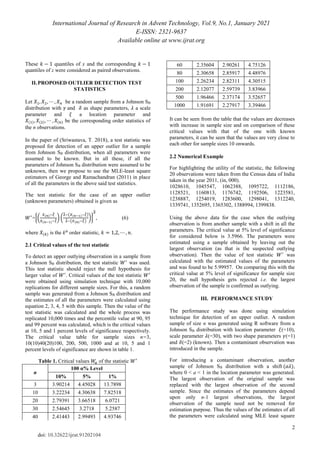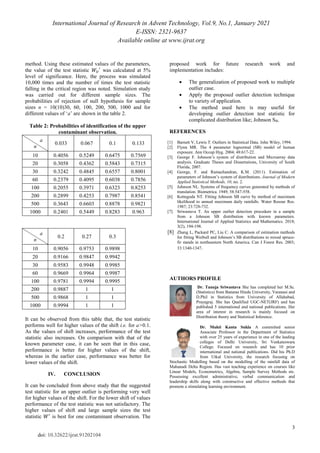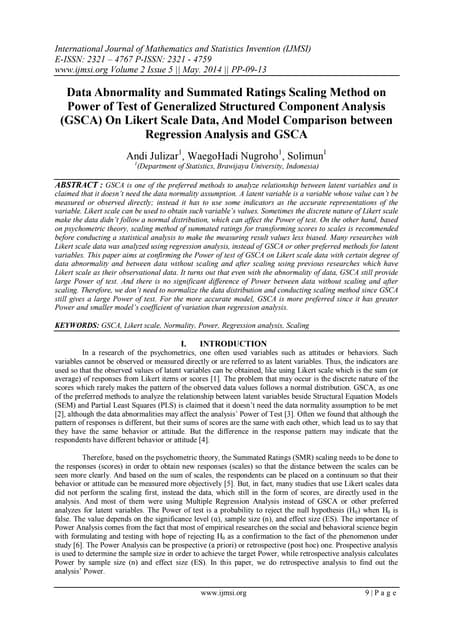PublicitéProchain SlideShareB025209013
Chargement dans ... 3
1 sur 3
Publicité

### 91202104

1. International Journal of Research in Advent Technology, Vol.9, No.1, January 2021 E-ISSN: 2321-9637 Available online at www.ijrat.org 1 doi: 10.32622/ijrat.91202104 Tests for a Single Upper Outlier in a Johnson SB Sample with Unknown Parameters Tanuja Sriwastava, Mukti Kant Sukla Abstract: Outliers are unexpected observations, which deviate from the majority of observations. Outlier detection and prediction are challenging tasks, because outliers are rare by definition. A test statistic for single upper outlier is proposed and applied to Johnson SB sample with unknown parameters. The Johnson SB distribution have four parameters and is extremely flexible, which means that it can fit a wide range of distribution shapes. Because of its distributional shapes it has a variety of applications in many fields. The test statistic proposed for the case when parameters are known (Sriwastava, T., 2018) used here for developing the test statistic when parameters are unknown. Critical points were calculated for different sample sizes and for different level of significance. The performance of the test in the presence of a single upper outlier is investigated. One numerical example was given for highlighting the result. Keywords: Outlier, Johnson SB Distribution, Trimmed Sample, Critical Points, Simulation. I. INTRODUCTION The p.d.f of Johnson SB distribution with location parameter 𝜉, scale parameter 𝜆 and two shape parameters 𝛾 𝑎𝑛𝑑 𝛿 is given by 𝑓(𝑥; 𝜉, 𝜆, 𝛾 , 𝛿) = 𝛿 √2𝜋 𝜆 (𝜆−(𝑥−𝜉))(𝑥−𝜉) 𝑒𝑥𝑝 [− 1 2 {𝛾 + 𝛿 ln ( 𝑥−𝜉 𝜆−(𝑥−𝜉) )} 2 ], (1) 𝜉 ≤ 𝑥 ≤ 𝜉 + 𝜆, 𝛿 > 0, −∞ < 𝛾 < ∞, 𝜆 > 0, − ∞ < 𝜉 < ∞. This distribution is extremely flexible, which means that it can fit a wide range of distribution shapes. Because of its distributional shapes it has a variety of application in many fields like human exposure (Flynn, 2004), forestry data (Zang et. al, 2003), rainfall data (Kotteguda, 1987) and manay more because of its flexible nature. One of the most important application of this distribution is in the complex data set like microarray data analysis (Florence George, 2007) etc. The detection of outliers in such situations are considerably important. A number of test statistic have been proposed for several distributions when their parameters are known (Barnett & Lewis, 1994). A test statistic has been proposed for the Johnson SB distribution when parameters are known by (Sriwastava, T., 2018) in one of her research paper. But in practice, no parameter would be known. In such situations, estimates of all the parameters are used in the construction of a test statistic for detection of outliers in a sample from a Johnson SB distribution. Then its critical values and the corresponding performance study were done by simulation technique. Since the study is about outlying observation, the entire sample should not be considered for the estimation of all the parameters. Hence, the estimates given by George and Ramachandran (2011) are used as estimates of all the parameters, using a trimmed sample obtained after removing the suspected outlying observation(s). The estimates of the parameters of this distribution as given by George & Ramachandran (2011) using maximum likelihood least square method was considered are as follows. 𝛾 ̂ = − 𝛿 ∑ 𝑔( 𝑥𝑖−𝜉 𝜆 ) 𝑛−1 𝑖=1 𝑛−1 = −𝛿𝑔̅ . (2) 𝛿 ̂2 = 𝑛−1 ∑ [𝑔( 𝑥𝑖−𝜉 𝜆 )] 2 𝑛−1 𝑖=1 − 1 𝑛−1 [∑ 𝑔( 𝑥𝑖−𝜉 𝜆 ) 𝑛−1 𝑖=1 ] 2 = 1 𝑣𝑎𝑟(𝑔) . (3) where, 𝑔 ( 𝑥−𝜉 𝜆 ) = log ( 𝑥−𝜉 𝜆−(𝑥−𝜉) ), 𝑔̅ is the mean and 𝑣𝑎𝑟(𝑔) is the variance of the values of g defined here. The estimates of 𝜆 and 𝜉 were as follows; 𝜆 ̂ = (𝑛−1) ∑ 𝑥𝑖 𝑛−1 𝑖=1 𝑓−1( 𝑧𝑖−𝛾 𝛿 )−∑ 𝑓−1( 𝑧𝑖−𝛾 𝛿 ) 𝑛−1 𝑖=1 ∑ 𝑥𝑖 𝑛−1 𝑖=1 (𝑛−1) ∑ [𝑓−1( 𝑧𝑖−𝛾 𝛿 )] 2 𝑛−1 𝑖=1 −[∑ 𝑓−1( 𝑧𝑖−𝛾 𝛿 ) 𝑛−1 𝑖=1 ] 2 .(4) 𝜉 ̂ = 𝑥̅ − 𝜆∗ 𝑚𝑒𝑎𝑛 [𝑓−1 ( 𝑧−𝛾 𝛿 )] , (5) where z= 𝑥−𝜉 𝜆 is a standard normal variate. Hence, the quantiles of x and the corresponding quantiles of z can be considered as paired observations. When there were 100 or more x values, the percentiles 1 through 99 were considered, while for k number of data points of x, where k is less than 100, 𝑘 − 1 quantiles of x were considered. Manuscript revised on January 29, 2021 and published on February 10, 2021 Dr. Tanuja Sriwastava, Assistant Professor, Department of Statistics, Sri Venkateswara College, University of Delhi. Email ID: tanujastat24@gmail.com, Dr. Mukti Kanta Sukla, Associate Professor, Department of Statistics, Sri Venkateswara College, University of Delhi. Email ID: suklamk@gmail.com
2. International Journal of Research in Advent Technology, Vol.9, No.1, January 2021 E-ISSN: 2321-9637 Available online at www.ijrat.org 2 doi: 10.32622/ijrat.91202104 These 𝑘 − 1 quantiles of x and the corresponding 𝑘 − 1 quantiles of z were considered as paired observations. II.PROPOSED OUTLIER DETECTION TEST STATISTICS Let 𝑋1, 𝑋2, ⋯ , 𝑋𝑛 be a random sample from a Johnson SB distribution with 𝛾 and 𝛿 as shape parameters, 𝜆 a scale parameter and 𝜉 a location parameter and 𝑋(1), 𝑋(2), ⋯ , 𝑋(𝑛) be the corresponding order statistics of the n observations. In the paper of (Sriwastava, T. 2018), a test statistic was proposed for detection of an upper outlier for a sample from Johnson SB distribution, when all parameters were assumed to be known. But in all these, if all the parameters of Johnson SB distribution were assumed to be unknown, then we propose to use the MLE-least square estimators of George and Ramachandran (2011) in place of all the parameters in the above said test statistics. The test statistic for the case of an upper outlier (unknown parameters) obtained is given as 𝑊′ ={( 𝑋(𝑛)−𝜉 ̂ 𝑋(𝑛−1)−𝜉 ̂) ( 𝜆 ̂−(𝑋(𝑛−1)−𝜉 ̂) 𝜆 ̂−(𝑋(𝑛)−𝜉 ̂) )} 𝛿 ̂ , (6) where 𝑋(𝑘) is the kth order statistic, 𝑘 = 1,2, ⋯ , 𝑛. 2.1 Critical values of the test statistic To detect an upper outlying observation in a sample from a Johnson SB distribution, the test statistic 𝑊′ was used. This test statistic should reject the null hypothesis for larger value of 𝑊′ . Critical values of the test statistic 𝑊′ were obtained using simulation technique with 10,000 replications for different sample sizes. For this, a random sample was generated from a Johnson SB distribution and the estimates of all the parameters were calculated using equation 2, 3, 4, 5 with this sample. Then the value of the test statistic was calculated and the whole process was replicated 10,000 times and the percentile value at 90, 95 and 99 percent was calculated, which is the critical values at 10, 5 and 1 percent levels of significance respectively. The critical value table for sample sizes n=3, 10(10)40(20)100, 200, 500, 1000 and at 10, 5 and 1 percent levels of significance are shown in table 1. Table 1. Critical values 𝑊 𝛼 of the statistic 𝑊′ n 100 α% Level 10% 5% 1% 3 3.90214 4.45028 13.7898 10 3.22234 4.30638 7.82518 20 2.79391 3.66518 6.0721 30 2.54645 3.2718 5.2587 40 2.41443 2.99493 4.93746 60 2.35604 2.90261 4.75126 80 2.30658 2.85917 4.48976 100 2.26234 2.82311 4.30515 200 2.12077 2.59739 3.83966 500 1.96466 2.37174 3.52657 1000 1.91691 2.27917 3.39466 It can be seen from the table that the values are decreases with increase in sample size and on comparison of these critical values with that of the one with known parameters, it can be seen that the values are very close to each other for sample sizes 10 onwards. 2.2 Numerical Example For highlighting the utility of the statistic, the following 20 observations were taken from the Census data of India taken in the year 2011, (in, 000). 1028610, 1045547, 1062388, 1095722, 1112186, 1128521, 1160813, 1176742, 1192506, 1223581, 1238887, 1254019, 1283600, 1298041, 1312240, 1339741, 1352695, 1365302, 1388994, 1399838. Using the above data for the case when the outlying observation is from another sample with a shift in all the parameters. The critical value at 5% level of significance for considered below is 3.5966. The parameters were estimated using a sample obtained by leaving out the largest observation (as that is the suspected outlying observation). Then the value of test statistic 𝑊′ was calculated with the estimated values of the parameters and was found to be 5.99957. On comparing this with the critical value at 5% level of significance for sample size 20, the null hypothesis gets rejected i.e. the largest observation of the sample is confirmed as outlying. III. PERFORMANCE STUDY The performance study was done using simulation technique for detection of an upper outlier. A random sample of size n was generated using R software from a Johnson SB distribution with location parameter 𝜉(=10), scale parameter 𝜆(=30), with two shape parameters 𝛾(=1) and 𝛿(=2) (known). Then a contaminant observation was introduced in the sample. For introducing a contaminant observation, another sample of Johnson SB distribution with a shift (𝑎𝜆), where 0 < a < 1 in the location parameter was generated. The largest observation of the original sample was replaced with the largest observation of the second sample. Since the estimates of the parameters depend upon only n-1 largest observations, the largest observation of the sample need not be removed for estimation purpose. Thus the values of the estimates of all the parameters were calculated using MLE least square
3. International Journal of Research in Advent Technology, Vol.9, No.1, January 2021 E-ISSN: 2321-9637 Available online at www.ijrat.org 3 doi: 10.32622/ijrat.91202104 method. Using these estimated values of the parameters, the value of the test statistic 𝑊𝑈 ′ was calculated at 5% level of significance. Here, the process was simulated 10,000 times and the number of times the test statistic falling in the critical region was noted. Simulation study was carried out for different sample sizes. The probabilities of rejection of null hypothesis for sample sizes n = 10(10)30, 60, 100, 200, 500, 1000 and for different values of ‘a’ are shown in the table 2. Table 2: Probabilities of identification of the upper contaminant observation. a n 0.033 0.067 0.1 0.133 10 0.4056 0.5249 0.6475 0.7569 20 0.3058 0.4362 0.5843 0.7315 30 0.3242 0.4845 0.6557 0.8001 60 0.2379 0.4095 0.6038 0.7856 100 0.2055 0.3971 0.6323 0.8253 200 0.2899 0.4253 0.7987 0.8541 500 0.3643 0.6603 0.8878 0.9821 1000 0.2401 0.5449 0.8283 0.963 a n 0.2 0.27 0.3 10 0.9056 0.9753 0.9898 20 0.9166 0.9847 0.9942 30 0.9583 0.9948 0.9985 60 0.9669 0.9964 0.9987 100 0.9781 0.9994 0.9995 200 0.9887 1 1 500 0.9868 1 1 1000 0.9994 1 1 It can be observed from this table that, the test statistic performs well for higher values of the shift i.e. for a>0.1. As the values of shift increases, performance of the test statistic also increases. On comparison with that of the known parameter case, it can be seen that in this case, performance is better for higher values of the shift, whereas in the earlier case, performance was better for lower values of the shift. IV. CONCLUSION It can be concluded from above study that the suggested test statistic for an upper outlier is performing very well for higher values of the shift. For the lower shift of values performance of the test statistic was not satisfactory. The higher values of shift and large sample sizes the test statistic 𝑊′ is best for one contaminant observation. The proposed work for future research work and implementation includes:  The generalization of proposed work to multiple outlier case.  Apply the proposed outlier detection technique to variety of application.  The method used here is may useful for developing outlier detection test statistic for complicated distribution like; Johnson SB. REFERENCES  Barnett V, Lewis T. Outliers in Statistical Data. John Wiley, 1994.  Flynn MR. The 4 parameter lognormal (SB) model of human exposure. Ann Occup Hyg. 2004; 48:617-22.  George F. Johnson’s system of distribution and Microarray data analysis. Graduate Theses and Dissertations, University of South Florida, 2007.  George, F. and Ramachandran, K.M. (2011). Estimation of parameters of Johnson’s system of distributions. Journal of Modern Applied Statistical Methods, 10, no. 2.  Johnson NL. Systems of frequency curves generated by methods of translation. Biometrica. 1949; 58:547-558.  Kottegoda NT. Fitting Johnson SB curve by method of maximum likelihood to annual maximum daily rainfalls. Water Resour Res. 1987; 23:728-732.  Sriwastava T. An upper outlier detection procedure in a sample from a Johnson SB distribution with known parameters. International Journal of Applied Statistics and Mathematics. 2018; 3(2), 194-198.  Zhang L, Packard PC, Liu C. A comparison of estimation methods for fitting Weibull and Johnson’s SB distributions to mixed spruce- fir stands in northeastern North America. Can J Forest Res. 2003; 33:1340-1347. AUTHORS PROFILE Dr. Tanuja Sriwastava She has completed her M.Sc. (Statistics) from Banaras Hindu University, Varanasi and D.Phil in Statistics from University of Allahabad, Prayagraj. She has Qualified UGC-NET(JRF) and has published 5 international and national publications. Her area of interest in research is mainly focused on Distribution theory and Statistical Inference. Dr. Mukti Kanta Sukla A committed senior Associate Professor in the Department of Statistics with over 25 years of experience in one of the leading colleges of Delhi University, Sri Venkateswara College. Focused on research and has 10 prior international and national publications. Did his Ph.D from Utkal University, the research focusing on Stochastic Modelling based on the modelling of the rainfall data of Mahanadi Delta Region. Has vast teaching experience on courses like Linear Models, Econometrics, Algebra, Sample Survey Methods etc. Possessing excellent administrative, verbal communication and leadership skills along with constructive and effective methods that promote a stimulating learning environment.
Publicité